# How to Build an Artificial Neural Network Basic Algorithm

We will be going through the ground of the neural network algorithm structure by building a color shade predictor, using artificial single layer architecture written in javascript, without any external libraries.

The goal of this publication is to briefly introduce you to the neural network algorithm structure without deep dive into the optimization potential, different strategies, and advanced examples of neural network structure. Surprisingly for following along, there is not so much math needed if you can add, subtract and multiply you are good to go, it will just be applied to matrixes and we will write everything by hand and without external dependencies to understand and control every part of the logic.

You can see the algorithm that we will build in action https://albertbol.github.io/nn-and-ga-example/ on the “Neural network classifier” tab, click “Train”:

And then pick RGB values to test prediction:

## A little bit of terminology

Before we take a look at algorithm steps, it would be easier to understand what we are doing by looking at the artificial neural network architecture presentation:

Let's take a look at all layers first in a nutshell.

The input layer is a single layer but can have a lot of nodes depending on the input itself, in this case, it's 3 nodes. Nodes are representing input values, let's say in our example it's R(red), G(green), B(blue) and are always normalized from 0 to 1 range. In our case max value is 255, so we will divide RGB by 255 to get the percentual normalized input value.

A hidden layer can be multi-layer, can have a different count of nodes. If the data is linearly separable hidden layers can be skipped. Less complex data can have 1 to 2 layers, large dimensions can have 3 to 5 hidden layers. The count of nodes usually is between the size of input and output nodes, this formula can be used: sqrt(input layer nodes * output layer nodes).

In the output layer, we want to get answers, nodes are representing classification, for example, we will be using colors, so each node will present a color like black and will have a number between 0 and 1, which will present the probability of prediction, so the size of output layer nodes is the count of the colors we want to predict.

Then you can see there are weights between input and hidden, hidden and output layers. In a nutshell, it's just a randomized number between 0 and 1 for each node connection, that will be multiplied on the previous node value it's connected to, exactly as a weight adjusting it to the right direction, and weights itself will be balanced due training cycles, its a core of the whole algorithm idea.

Now we will take the rest of the terminology and we are ready to go, its bias, activation function, gradient calculation, and learning rate.

Bias is a value that will be added to the node input after weight multiplication applied, adding a constant value is preventing it from showing the inaccurate result and helps activation function to make the right decision, let us imagine our input is 0, then no matter which weight we have it still will be 0 after multiplication, so all next step will lead to 0, but we want to be more precise for better weights adjustment in the training phase.

The activation function is like a switcher, it will take the previous node value after weights multiplication and bias addition and decide whether it's on the left or right side of the function output range. In our case, we will be using the ReLU activation function which we will discuss later in the article.

Gradient descent calculation is used in the training cycle only, it will be adjusting weights and bias. Its goal is to find a local function minimum, by taking repeated steps in the opposite direction of the gradient of the function at the current point, because this is the direction of steepest descent.

It's like going down the mountain taking a shortcut gradually until reaching the lowest point.

Learning rate is basically a determination of the step in the gradient descent function. We are multiplying weight values on the gradient to adjust them. To avoid overfitting and make it more precise we are multiplying gradient values on learning rate before, often in the range between 0.0 and 1.0 for balancing.

Now when we are done with the heaviest part, let's discuss how all this is applied in the algorithm itself.

## Let's start building

We want to pass 3 values (RGB) and get back a color prediction. In this case, in our input layer, we will have 3 input nodes, representing red, green, blue values from 0–255.

Let's skip hidden and take the output layer now, we will want to predict 12 color shades, so we will have 12 nodes representing each color.

So now we can count our hidden layer using the formula mentioned above sqrt(input layer nodes * output layer nodes), sqrt(3 * 12) = 6.

Now when we have our neural network architecture ready, there are 2 phases we need to handle: training and predicting. Let's write code and learn by example.

## Matrix helper class

As I said before there will be only additions, subtraction, and multiplying, but as we have arrays of numbers it's easier to present them as matrixes, so all those operations we will apply to them. We will be using a small homemade Matrix class with functions that we will need through the process it's very straightforward and we will not focus on explaining every function there, instead, you can take a look at gist: https://gist.github.com/Albertbol/e729220758f4c70fb38bef622e802c37

## Neural network class constructor setup

Let's prepare our NeuralNetwork class that will help us perform all actions to reach the result, we start from settings values we need in a constructor.

We pass input nodes, which is 3 for our situation, hidden nodes we calculated 6, the output is 12 color shades. For the learning rate, we can use the value 0.01. For bias, we will use static number 1. Training cycles will decide how many iterations we will perform, the number of cycles has no strict rule and depends on the quality of training data, size of nodes, and layers. It's possible to underfit or overfit the algorithm so it should be tested to find out the best rate for a specific case, we will choose 50 000. Normalizer is the maximum value in input, in our case 255, to be used later for value normalization to percentual.

The values we have chosen can be changed and should be to find out the best ones for this model, the only static values are normalized and input nodes size, everything else can be adjusted even output if we add more than 12 color shades.

Now we need to create weights matrixes for input to hidden and hidden to output connections. Where rows are next layer node count and columns previous layers node count. Starting weights values are complete random, there are different strategies on how to generate them from the beginning to increase performance that you can dive deeper later on, but random is used quite often, so we pass the random normalized option. It's called randomly normalized, but not just random, because we actually create 4 random numbers and divide them by 4 to get the average number, so we have a better, smoother random number distribution, which will fit better for adjustments.

Let's create bias matrixes for input and hidden layers. The row size is a layer node count and the column is 1, filled with static bias number which we configured as 1.

Great, 🎉 we are ready to write the main logic.

## Prediction

We will start from prediction because even if it sounds surprising it's an easier operation than training and the training cycle has a prediction inside of it.

Prediction in the neural network is called feeding forward because we are pushing input values through the nodes and all layers until we reach the output. We can present it like this:

Where input array argument is for example [255,255,255] RGB values. Then we normalize it, which will just divide input values on max value (255), to get percentual normalization:

Now we are ready to create a matrix from the input array, you can check the gist in the matrix helper class section for the operation details mentioned above. After that we are passing the matrix for this layer forward to apply weights, bias, and activation function, we use calculateFF function for this, first, we do that for the input layer, then hidden and we get output, if we would have more hidden layers, it would continue until reaching the output.

CalculateFF function is multiplying weights and input matrix to get the next layer output, after that, we are adding our bias and applying activation function ReLU to each value in the output matrix.

It's as simple as that, any number over 0 will be just returned as it is, otherwise, it will be 0.0.

Now we have our output logic and the whole neural network feeding forward process described, because we are using matrixes we don't care that much how many nodes we have and if we have more layers in the hidden part we can just iterate through all of them and get our output, reusing the same logic.

## Training

Training is where all the “magic” happens 🧙‍♂️. And by magic we mean weights adjustment, but it's a multi-step process that we will go through.

We will be using here supervised learning, which means that we are providing input values with the right answer. The feeding forward algorithm gets the result and we adjust the weights accordingly.

The color shades that we want to predict are:

And training data looks like this:

We tell that if an input is RGB [0,0,0] it's a black color shade 100%. I was using this website to set up training data https://www.rapidtables.com/web/color/black-color.html and you can use the same training data from this gist.

Ok, we have everything to start training our model. Training data quality is very important it should contain possibly only the right answers to be precise, should be different to help to adjust flexible weights and there should be enough data also.

Let's take a look at our train function:

It's an iteration cycle that we configured in the class constructor above. You will see that we are picking random data from the training date array, we do that to prevent any kind of patterns and even pass the multiple times same information and so on.

Then we are just feeding forward input data to get the result and grabbing the correct value from the training data array.

Now we start a process called backpropagation, it's just the same thing as we did for feeding forward but now we will go backward to calculate the difference between correct value and output we got to adjust every weight in the network.

We will start by adjusting hidden to output layer weights. Let's calculate the error difference between the right values and those that we got, usually it's called delta error, by simply subtracting correct answers with output matrixes.

Now when we have output error delta matrix we can pass it to gradients descent co calculate gradient that can be used to adjust weights. The next step will be adjusting weights. We will take a look at those functions after we will get through the main logic in the training function.

Now when we balanced the hidden to output layer connection we can move backward to the next layer's connection, which is input to hidden. To do this we will be using weights that we adjusted in the previous step and transpose them because we are moving backward. Now we can calculate hidden error by multiplying transposed weights and output error. Now calculate gradient for hidden layer and adjust weights from input to hidden layer.

Ok, we went through the main steps, let’s now take a look at how actually calculate gradient descent function works.

We are taking an input argument that represents the layer we are adjusting. To calculate gradients we will be using the ReLU activation function again, but it will be derivative because again we are moving backward, which is very simple:

We take the input matrix and map each value with derivativeReLU. Then we are mapping each value to the delta error matrix that we passed as an argument. Now it's the last step to use learning rate, for preventing overfitting and make smaller steps, because we have a lot of different values and cycles, we want to make small adjustments that will fit all possible input and outputs, otherwise, we will be adjusting weights just for this specific input every time and our model, in the end, will be just trained to predict last input we have sent. We will map each gradient value multiplying by the learning rate.

Finally, let's take a look at the most important function adjust weights:

Input argument represents the layer that we are adjusting. We transpose it also because we are doing everything backward. Now we can get the weights delta by multiplying the gradient we passed on input. After that, we can adjust the weights and map them adding each delta weight, to get the new matrix with balance values. Now we want to adjust bias also, we take constant bias matrix for this layer connection passed as an argument and also add a gradient to it.

Training is done, it's a good idea to save some testing data, different from training one, but where you know the right answer to see how well your model performs after training, to adjust for reaching right precision.

## Congratulations 🎉

You have gone through the whole neural network-building process and built your own color shade prediction algorithm. It's so much more to cover on this topic, but the main goal was to understand the concept and move forward diving deeper into the different strategies, the importance of each part, architecture itself, methods, and many more, but now we have the basics and it's much easier to learn further.

We have tried to build everything by ourselves to understand basics better, but in the programming world, we don't need to focus on re-building the cycle, again and again, better focus on new directions and reuse solution as much as possible, so of course, there are plenty of brilliant libraries that will take care of a lot of manual work for us, using better algorithms and strategies and lets us adjust small parts to get the best results, like TensorFlow.

You can see full neural-network class code here: https://gist.github.com/Albertbol/57f695a3a3cf7e4c73a4ce1b9d758094

I would thank and recommend the following sources to dive deeper into the subject and gain even stronger basics:

Coding train youtube channel Neural Networks — The Nature of Code playlist.
”Make Your Own Neural Network” book by Tariq Rashid.
3Blue1Brown “But what is a Neural Network? Deep learning 4 videos series.

Also, feel free to check out my article for genetic algorithms “How to build a genetic algorithm basic introduction” (https://medium.com/codex/how-to-build-a-genetic-algorithm-basic-introduction-c6a7cd503499)

--

--

--

## More from Alb Bolush

Web Developer

Love podcasts or audiobooks? Learn on the go with our new app.

## An introduction to Machine Learning## How to classify everyday images using Machine Learning?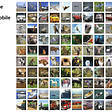## A sudden change to the encoder!## Segmentation of Brachial Plexus from Ultrasound Images using U-Net Architecture in Keras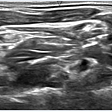## Detecting objective through Detectron2.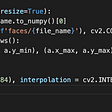## Online Shoppers Purchasing Intention with Machine Learning## PyTorch Scholarship Challenge from Facebook: I’m in!## OpenCV: Images HandlingWeb Developer

## ArcFace Project (Part 2/5)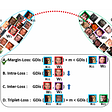## Classifying breeds deep learning approach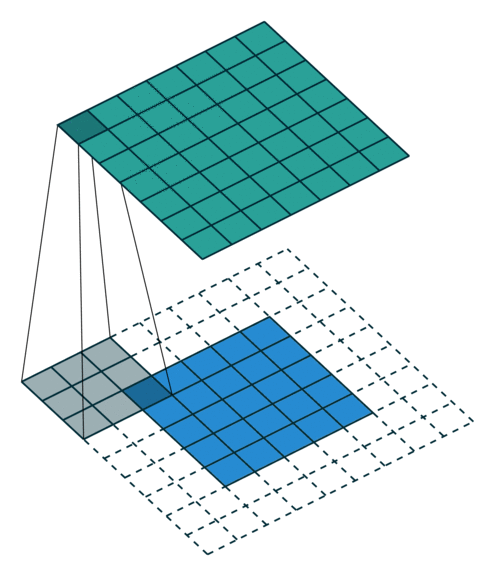## OOPS I deleted it Again !!!